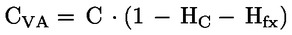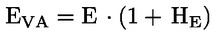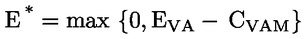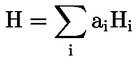In force

Version from: 01/01/2023
Amendments (2)
Search within this legal act

### Article 223 - Financial Collateral Comprehensive Method

Article 223

Financial Collateral Comprehensive Method

1.
In order to take account of price volatility, institutions shall apply volatility adjustments to the market value of collateral, as set out in Articles 224 to 227, when valuing financial collateral for the purposes of the Financial Collateral Comprehensive Method.

Where collateral is denominated in a currency that differs from the currency in which the underlying exposure is denominated, institutions shall add an adjustment reflecting currency volatility to the volatility adjustment appropriate to the collateral as set out in Articles 224 to 227.

In the case of OTC derivatives transactions covered by netting agreements recognised by the competent authorities under Chapter 6, institutions shall apply a volatility adjustment reflecting currency volatility when there is a mismatch between the collateral currency and the settlement currency. Even where multiple currencies are involved in the transactions covered by the netting agreement, institutions shall apply a single volatility adjustment.

2.

Institutions shall calculate the volatility-adjusted value of the collateral (CVA) they need to take into account as follows:where:

 C = the value of the collateral;
 HC = the volatility adjustment appropriate to the collateral, as calculated under Articles 224 and 227;
 Hfx = the volatility adjustment appropriate to currency mismatch, as calculated under Articles 224 and 227.

Institutions shall use the formula in this paragraph when calculating the volatility-adjusted value of the collateral for all transactions except for those transactions subject to recognised master netting agreements to which the provisions set out in Articles 220 and 221 apply.

3.

Institutions shall calculate the volatility-adjusted value of the exposure (EVA) they need to take into account as follows:where:

 E = the exposure value as would be determined under Chapter 2 or Chapter 3, as applicable, where the exposure was not collateralised;
 HE = the volatility adjustment appropriate to the exposure, as calculated under Articles 224 and 227.

In the case of OTC derivative transactions, institutions using the method laid down in Section 6 of Chapter 6 shall calculate EVA as follows:

EVA = E.
4.

For the purpose of calculating E in paragraph 3, the following shall apply:

(a)

for institutions calculating risk-weighted exposure amounts under the Standardised Approach, the exposure value of an off-balance sheet item listed in Annex I shall be 100 % of that item's value rather than the exposure value indicated in Article 111(1);

(b)

for institutions calculating risk-weighted exposure amounts under the IRB Approach, they shall calculate the exposure value of the items listed in Article 166(8) to (10) by using a conversion factor of 100 % rather than the conversion factors or percentages indicated in those paragraphs.

5.

Institutions shall calculate the fully adjusted value of the exposure (E*), taking into account both volatility and the risk-mitigating effects of collateral as follows:where:

 EVA = the volatility adjusted value of the exposure as calculated in paragraph 3;
 CVAM = CVA further adjusted for any maturity mismatch in accordance with the provisions of Section 5;

In the case of OTC derivative transactions, institutions using the methods laid down in Sections 3, 4 and 5 of Chapter 6 shall take into account the risk-mitigating effects of collateral in accordance with the provisions laid down in Sections 3, 4 and 5 of Chapter 6, as applicable.

6.
Institutions may calculate volatility adjustments either by using the Supervisory Volatility Adjustments Approach referred to in Article 224 or the Own Estimates Approach referred to in Article 225.

An institution may choose to use the Supervisory Volatility Adjustments Approach or the Own Estimates Approach independently of the choice it has made between the Standardised Approach and the IRB Approach for the calculation of risk-weighted exposure amounts.

However, where an institution uses the Own Estimates Approach, it shall do so for the full range of instrument types, excluding immaterial portfolios where it may use the Supervisory Volatility Adjustments Approach.

7.

Where the collateral consists of a number of eligible items, institutions shall calculate the volatility adjustment (H) as follows:where:

 ai = the proportion of the value of an eligible item i in the total value of collateral;
 Hi = the volatility adjustment applicable to eligible item i.